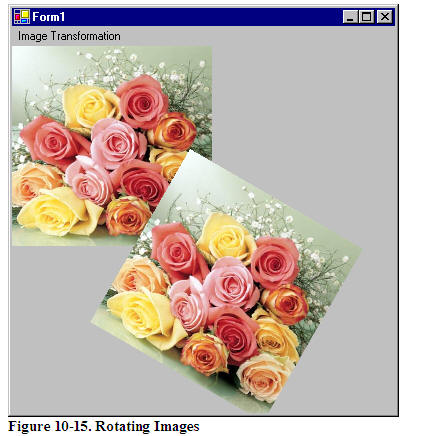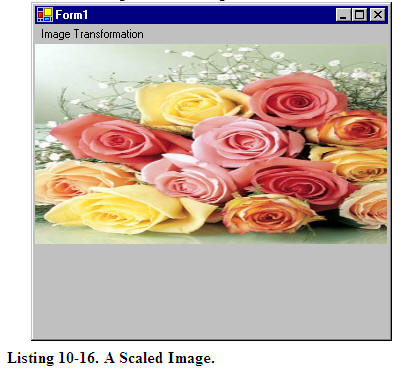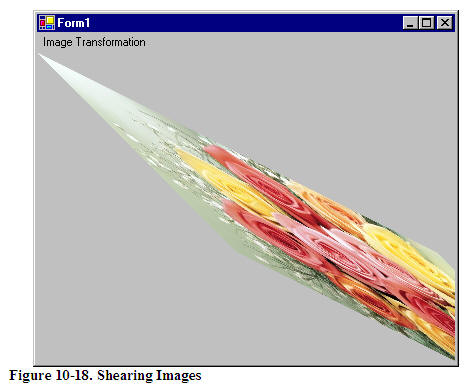# Image Transformation in C# with GDI+

Image transformation is exactly the same as any other transformation process. In this section we will see how to rotate, scale, translate, reflect, and shear images. We will create a Matrix object, set the transformation process by calling its methods, set the Matrix object as the Transform property or the transformation methods of the Graphics object, and call DrawImage.

Rotating images is similar to rotating other graphics. Listing 10.16 rotates an image. We create a Graphics object using the CreateGraphics method. Then we create a Bitmap object from a file and call the DrawImage method, which draws the image on the form. After that we create a Matrix object, call its Rotate method, rotate the image by 30 degrees, and apply the resulting matrix to the surface using the Transform property. Finally, we draw the image again using DrawImage.

Listing 10.16: Rotating images

private void RotationMenu_Click(object sender, System.EventArgs e)
{
Graphics g =
this
.CreateGraphics();
g.Clear(
this
.BackColor);
Bitmap curBitmap =
new
Bitmap(@"roses.jpg");
g.DrawImage(curBitmap, 0, 0, 200, 200);
// Create a Matrix object, call its Rotate method,
// and set it as Graphics.Transform
Matrix X = new
Matrix();
X.Rotate(30);
g.Transform = X;
// Draw image
g.DrawImage(curBitmap,
new
Rectangle(205, 0, 200, 200),
0, 0, curBitmap.Width,
curBitmap.Height,
GraphicsUnit.Pixel) ;
// Dispose of objects
curBitmap.Dispose();
g.Dispose();
}

Figure 10.17 shows the output from Listing 10.16. The first image is the original; the second image is rotated.Now let's apply other transformations. Replacing the Rotate method in Listing 10.16 with the following line scales the image:

X.Scale(2, 1, MatrixOrder.Append);

The scaled image is shown in Figure 10.18.Replacing the Rotate method in Listing 10.16 with the following line translates the image with 100 offset in the x- and y-directions:

X.Translate(100, 100);

The new output is shown in Figure 10.19.Replacing the Rotate method in Listing 10.16 with the following line shears the image:

X.Shear(2, 1);

The new output is shown in Figure 10.20.You have probably noticed that image transformation is really no different from the transformation of other graphics objects. I recommend that you download the source code samples from the book's Web site to see the detailed code listings.